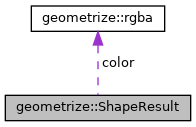Geometrize  1.0 C++ library for geometrizing images into geometric primitives
geometrize::ShapeResult Struct Reference

The ShapeResult struct is a container for info about a shape added to the model. More...

`#include <shaperesult.h>`

Collaboration diagram for geometrize::ShapeResult:[legend]

## Public Attributes

const double score

const geometrize::rgba color

const std::shared_ptr
< geometrize::Shape
shape

## Detailed Description

The ShapeResult struct is a container for info about a shape added to the model.

## Member Data Documentation

 const geometrize::rgba geometrize::ShapeResult::color
 const double geometrize::ShapeResult::score
 const std::shared_ptr geometrize::ShapeResult::shape

The documentation for this struct was generated from the following file:
• /home/travis/build/Tw1ddle/geometrize-lib-docs/geometrize/geometrize/geometrize/shaperesult.h